Go back to  'Decimals'

In this mini-lesson, we will explore how to add decimals by figuring out how to add and subtract decimals, as well as methods by which we can add decimals to whole numbers.

But here's an interesting bit of trivia: Did you know that the Greek scientist Archimedes (the same dude who ran through Syracuse without any clothes after he made one of the most groundbreaking discoveries in physics) was the person who invented decimal positional system. Decimal word comes from a Latin word known as decimus, meaning tenth. Hence, decimal system has 10 as its base and it is called a base 10-system.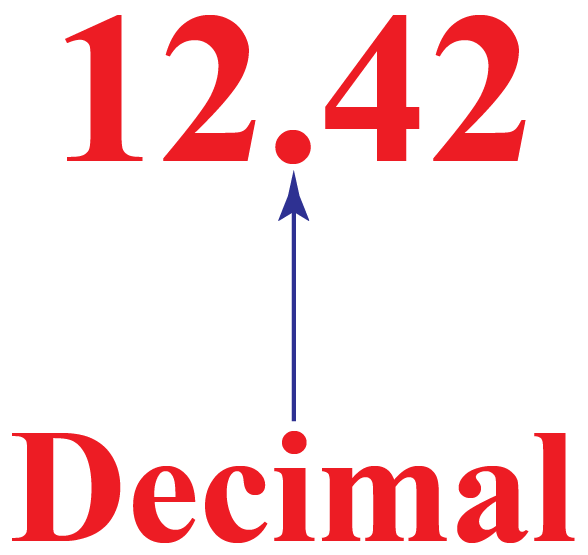## Lesson Plan

Before we proceed, let's have a decimal lesson recap.

It is possible to apply all the basic arithmetic operations, additionsubtraction, multiplication and division for decimals.

The addition of decimals is shown as below: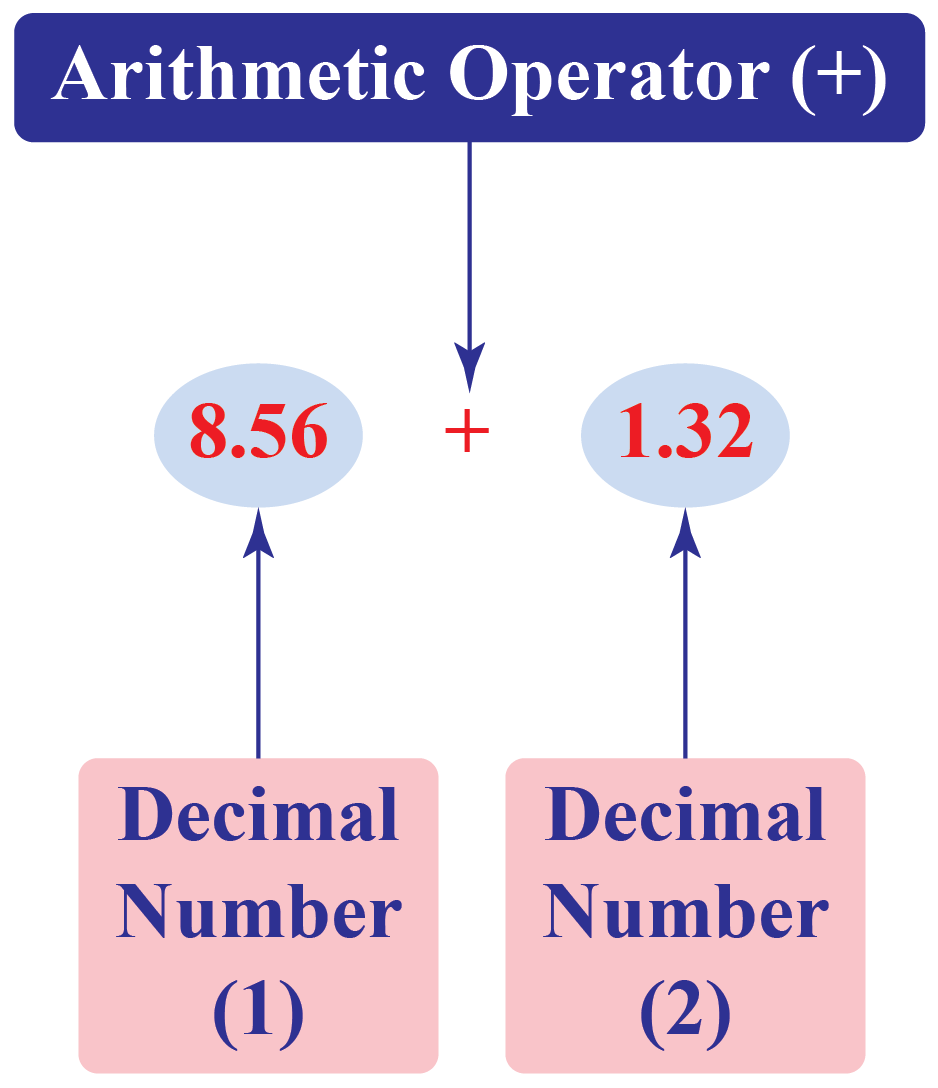The decimals are added using the following steps:

• Step 1: Write the numbers, such that the decimals are lined perfectly.
• Step 2: Write zeroes in the places wherever the length of decimal numbers is not same.
• Step 3: Now add the decimal numbers and find the output to addition.

Let's see an example for addition of decimals:

### Add $$8.6$$ and $$2.8$$

• Step 1: Write the numbers, such that the decimals are lined perfectly.

The numbers will be written as follows: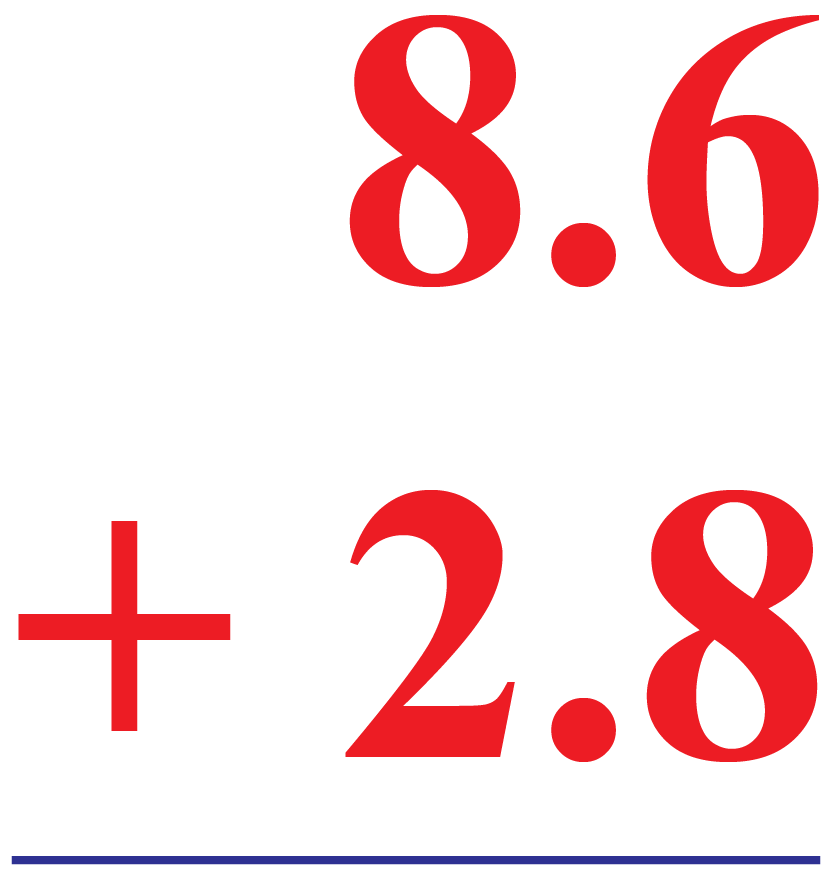• Step 2: Write zeroes in the places wherever the length of decimal numbers is not same.

This step does not apply in this case as length of the decimal numbers is same.

• Step 3: Now add the decimal numbers and find the output to addition.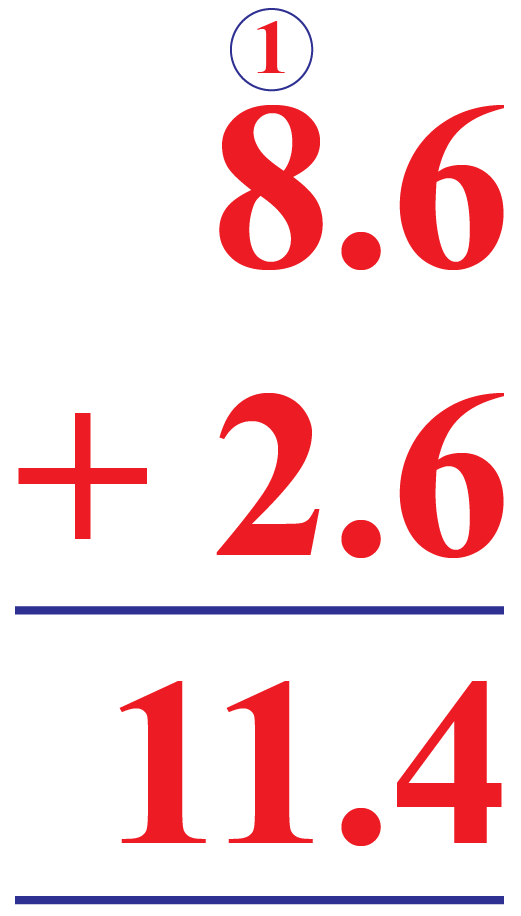The output obtained after addition of decimal numbers is, $$11.4$$.Tips and Tricks

• The addition of two decimal numbers can be treated as addition of two numbers initially and decimal can be placed in the end, to avoid any confusions.

## How to Add Decimals with Whole Numbers?

Let's see a few examples to add decimals with whole numbers.

### Add $$6.54$$ and $$4$$

• Step 1: Write the numbers, such that the decimals are lined perfectly.

The numbers will be written as follows: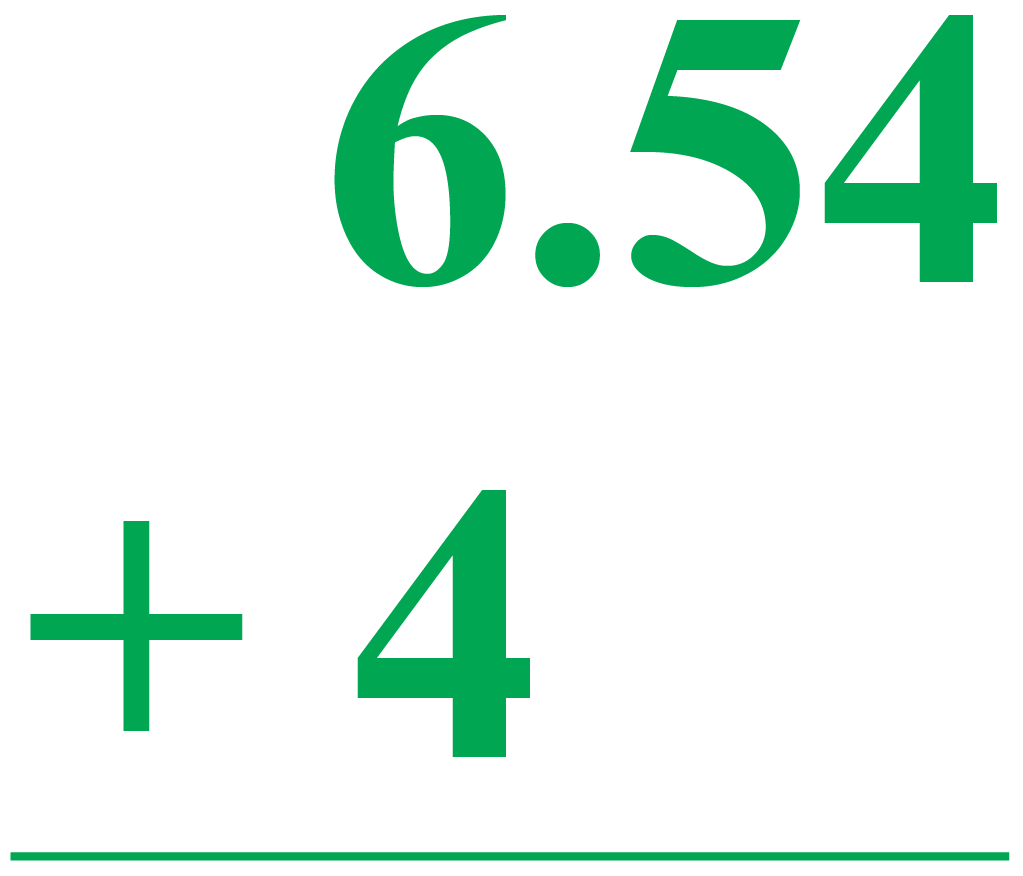• Step 2: Put zeroes equal to the other decimal number length, which makes it a decimal number.

As 4 is a whole number, two zeroes are added after the decimal, now the addition will now look like: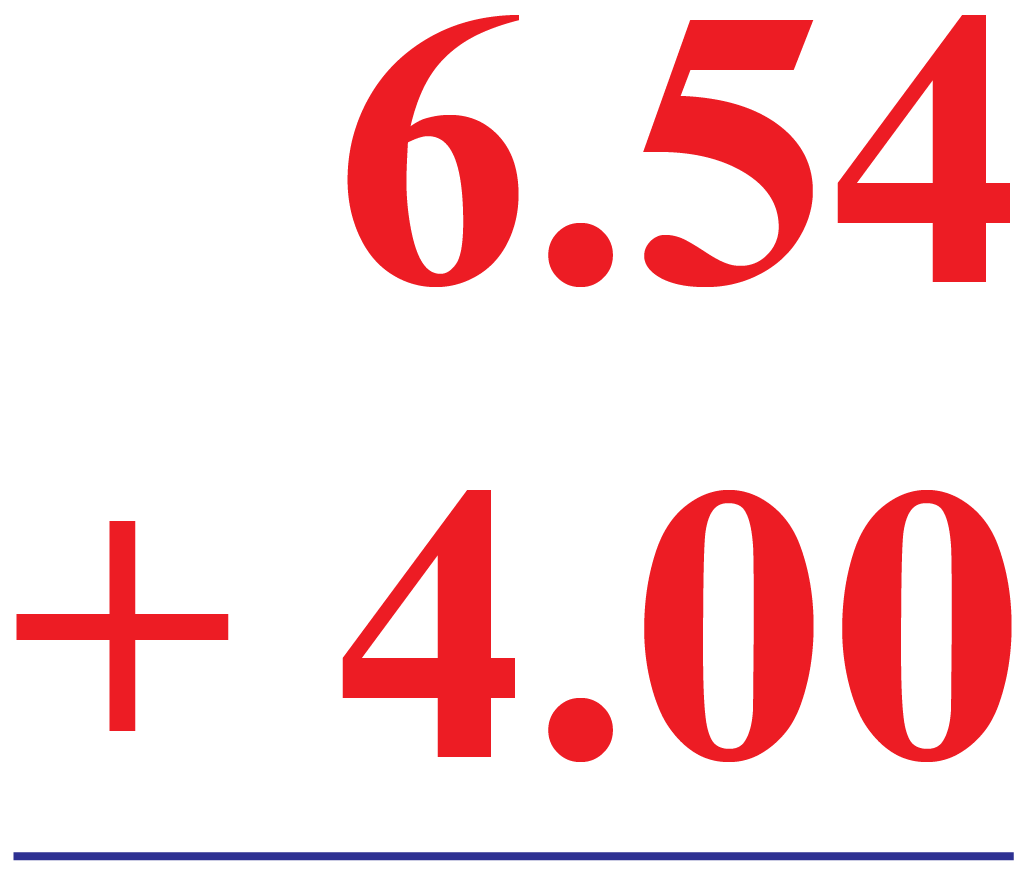• Step 3: Now add the decimal numbers and find the output to addition.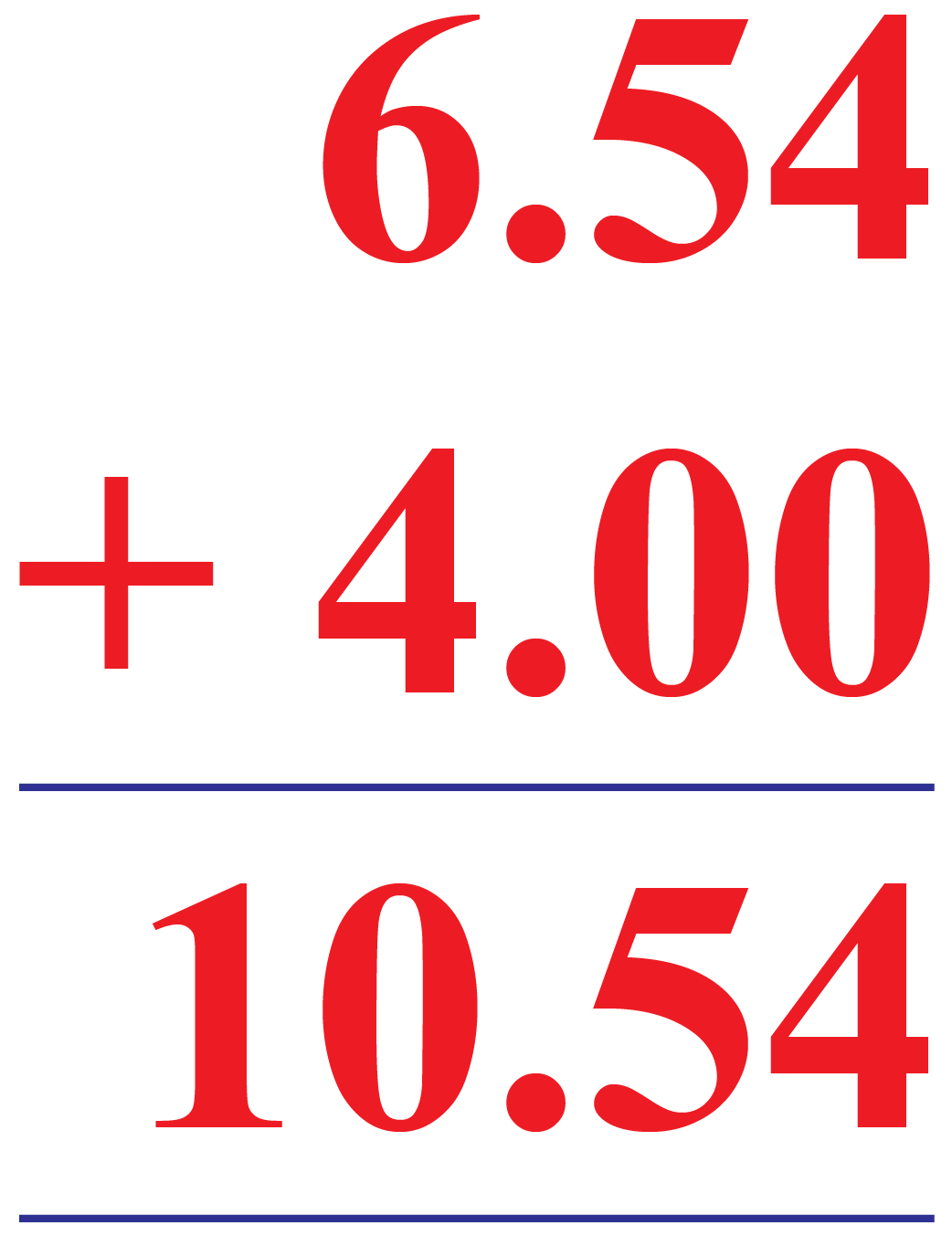The output obtained after addition of decimal numbers is, $$10.54$$.

### Add $$2.35$$ and $$5$$

• Step 1: Write the numbers, such that the decimals are lined perfectly.

The numbers will be written as follows: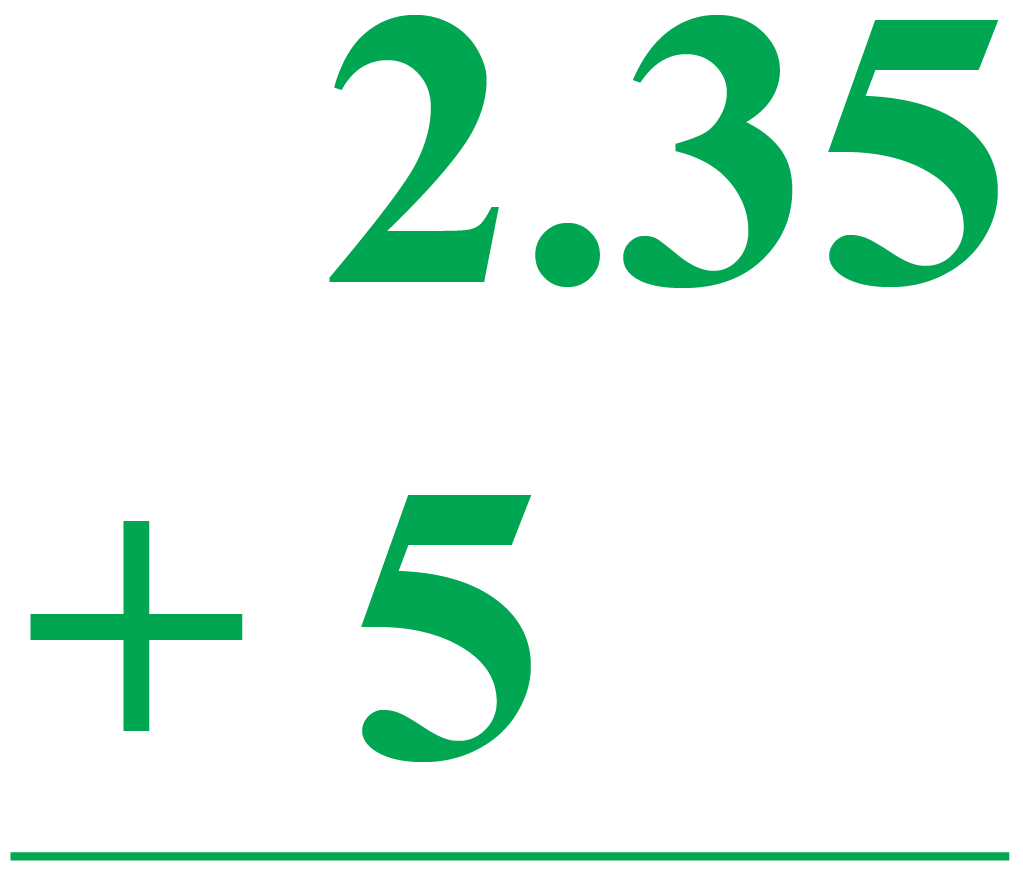• Step 2: Put zeroes equal to the other decimal number length, which makes it a decimal number.

As 5 is a whole number, two zeroes are added after the decimal, now the addition will now look like: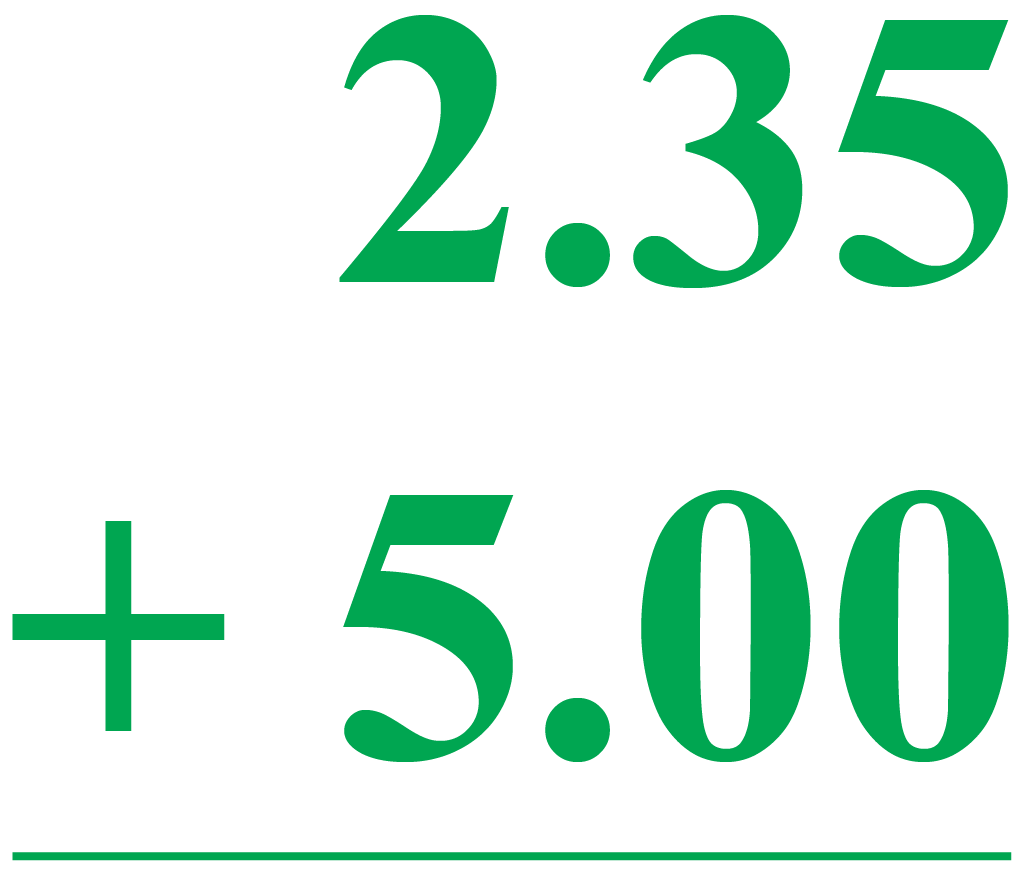• Step 3: Now add the decimal numbers and find the output to addition.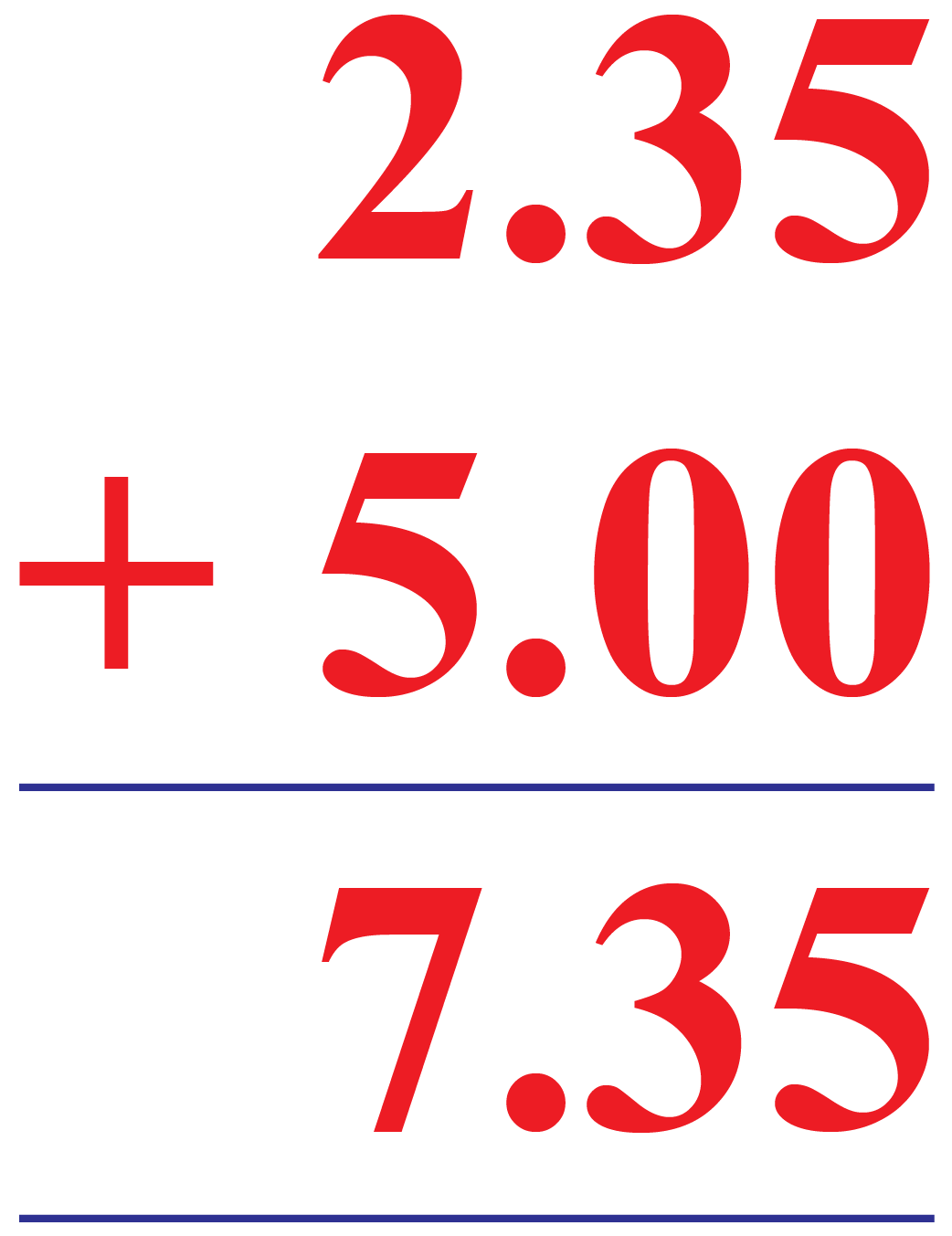The output obtained after addition of decimal numbers is, $$7.35$$.

### Add $$13.24$$ and $$25$$

• Step 1: Write the numbers, such that the decimals are lined perfectly.

The numbers will be written as follows: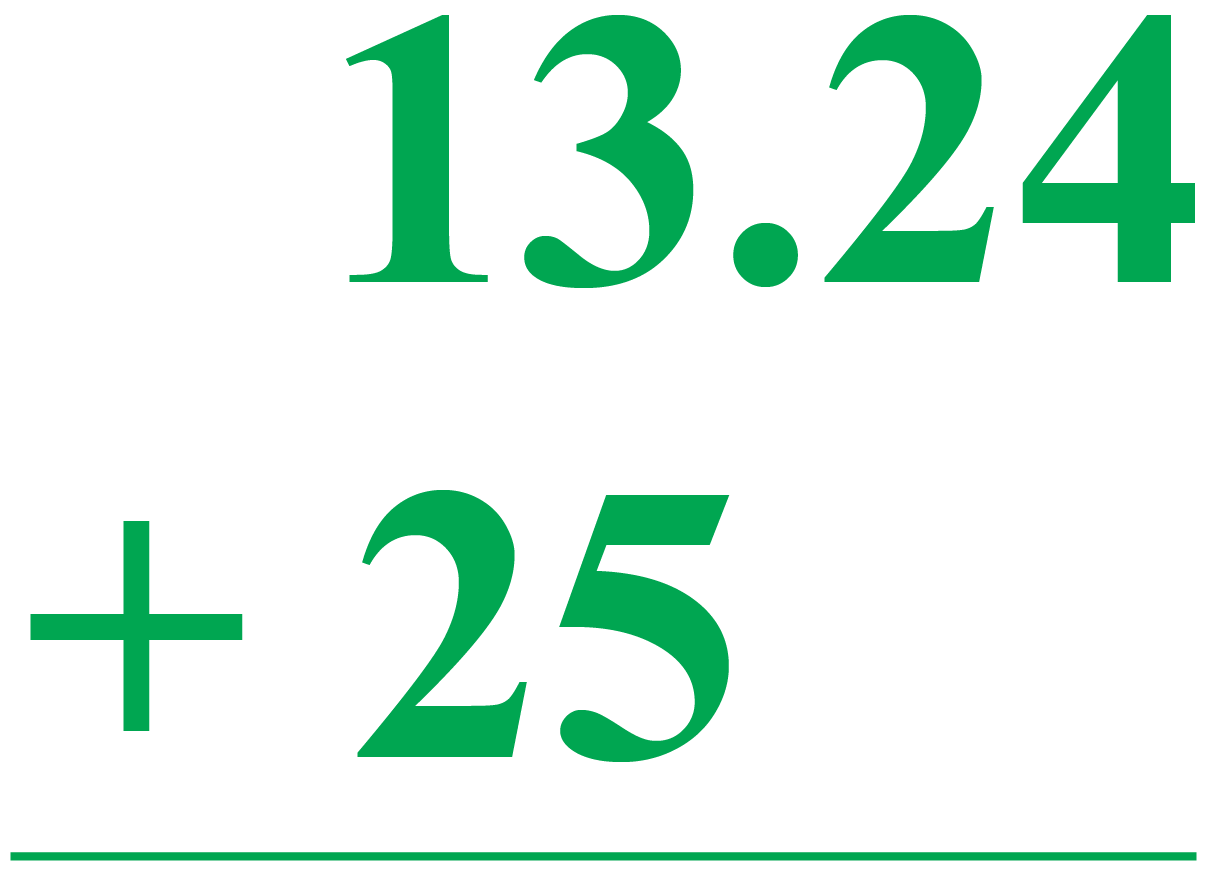• Step 2: Put zeroes equal to the other decimal number length, which makes it a decimal number.

As 25 is a whole number, two zeroes are added after the decimal, now the addition will now look like: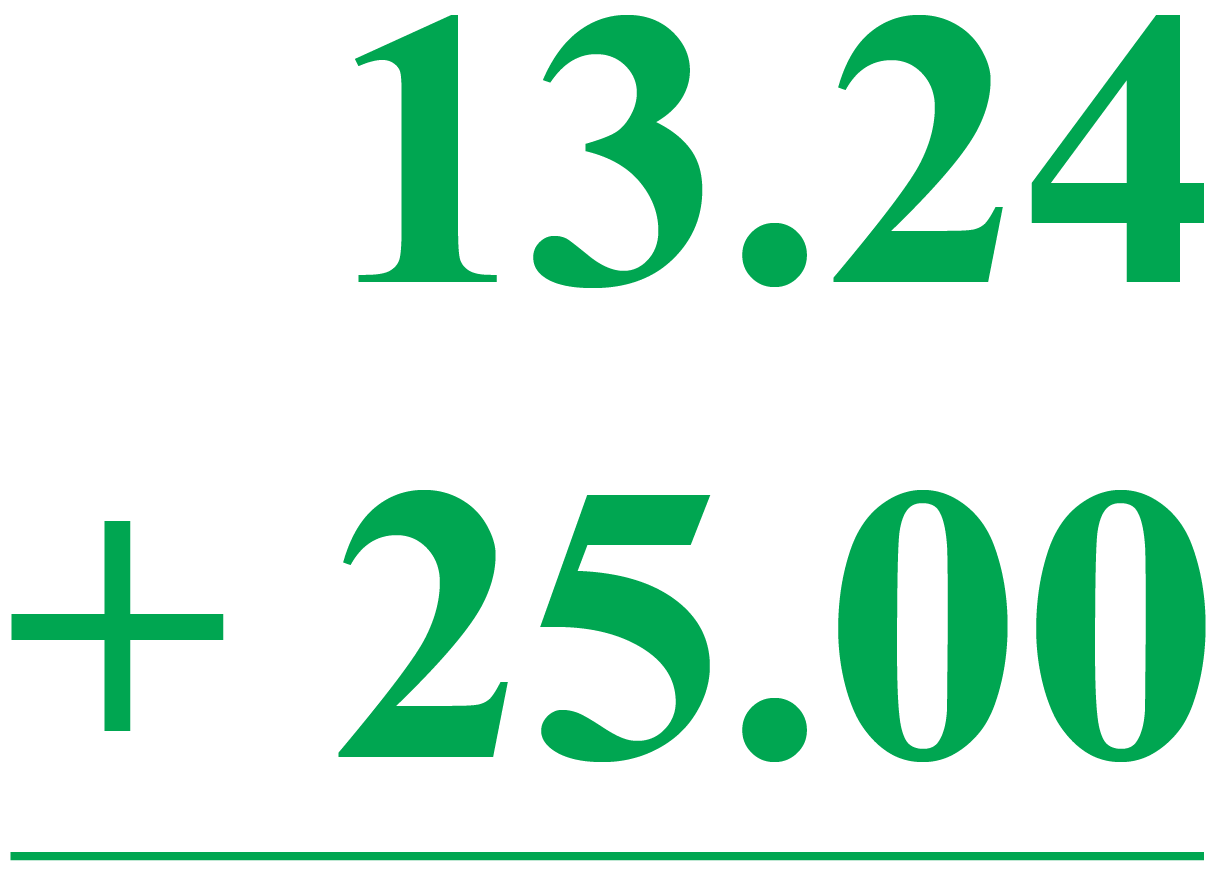• Step 3: Now add the decimal numbers and find the output to addition.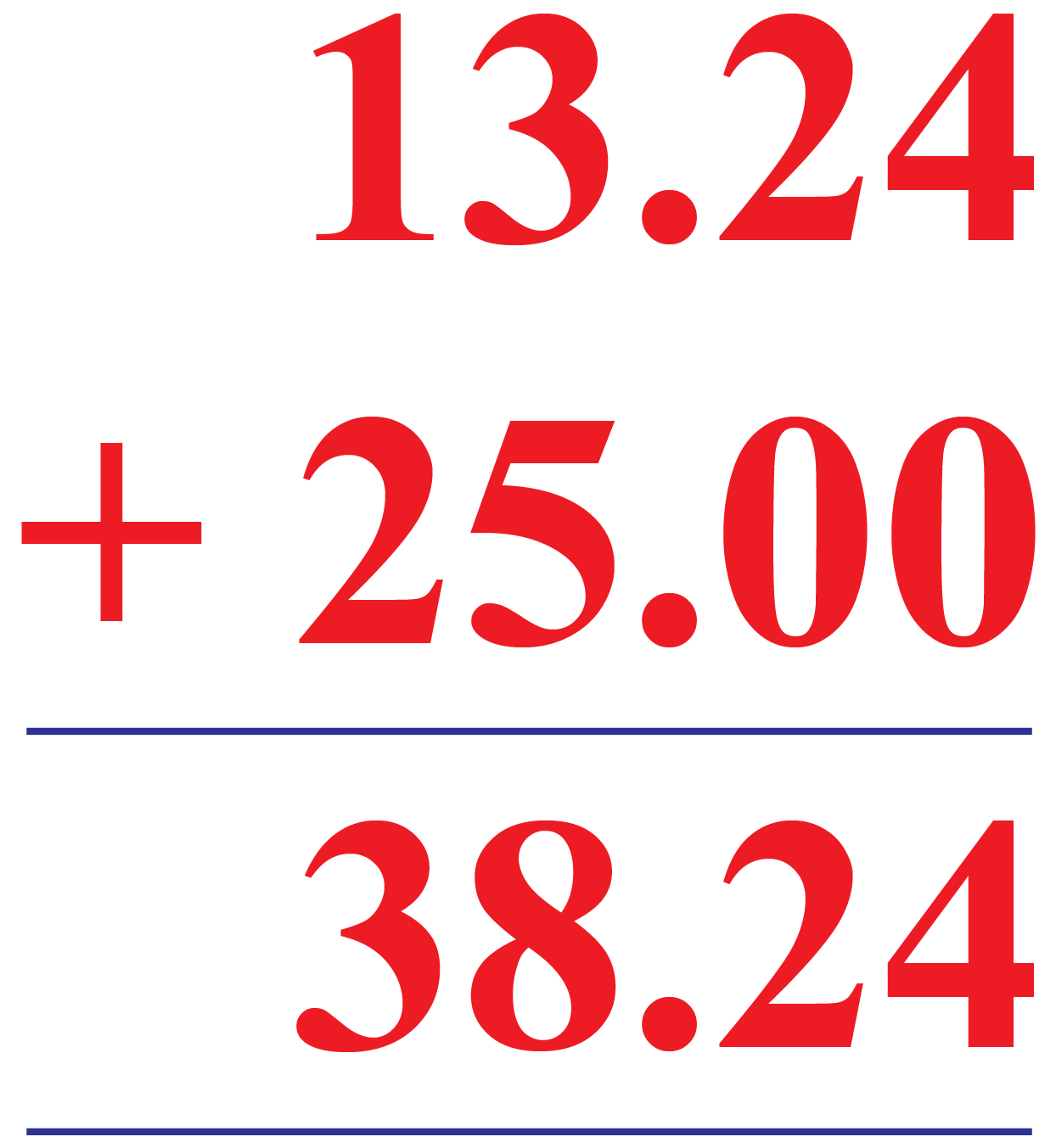The output obtained after addition of decimal numbers is, $$38.24$$.

Have a look at the simulation below:

## How to Add and Subtract Decimals?

We learnt adding decimals in above section, let's have a look at subtracting them too.

Subtracting Decimals

The decimals are subtracted using the following steps:

• Step 1: Write the numbers, such that the decimals are lined perfectly.
• Step 2: Write zeroes in the places wherever the length of decimal numbers is not same.
• Step 3: Now subtract the decimal numbers and find the output to subtraction.

Let's take a few examples to understand this concept better:

### Subtract $$12.11$$ from $$28.16$$

• Step 1: Write the numbers, such that the decimals are lined perfectly.

The numbers will be written as follows: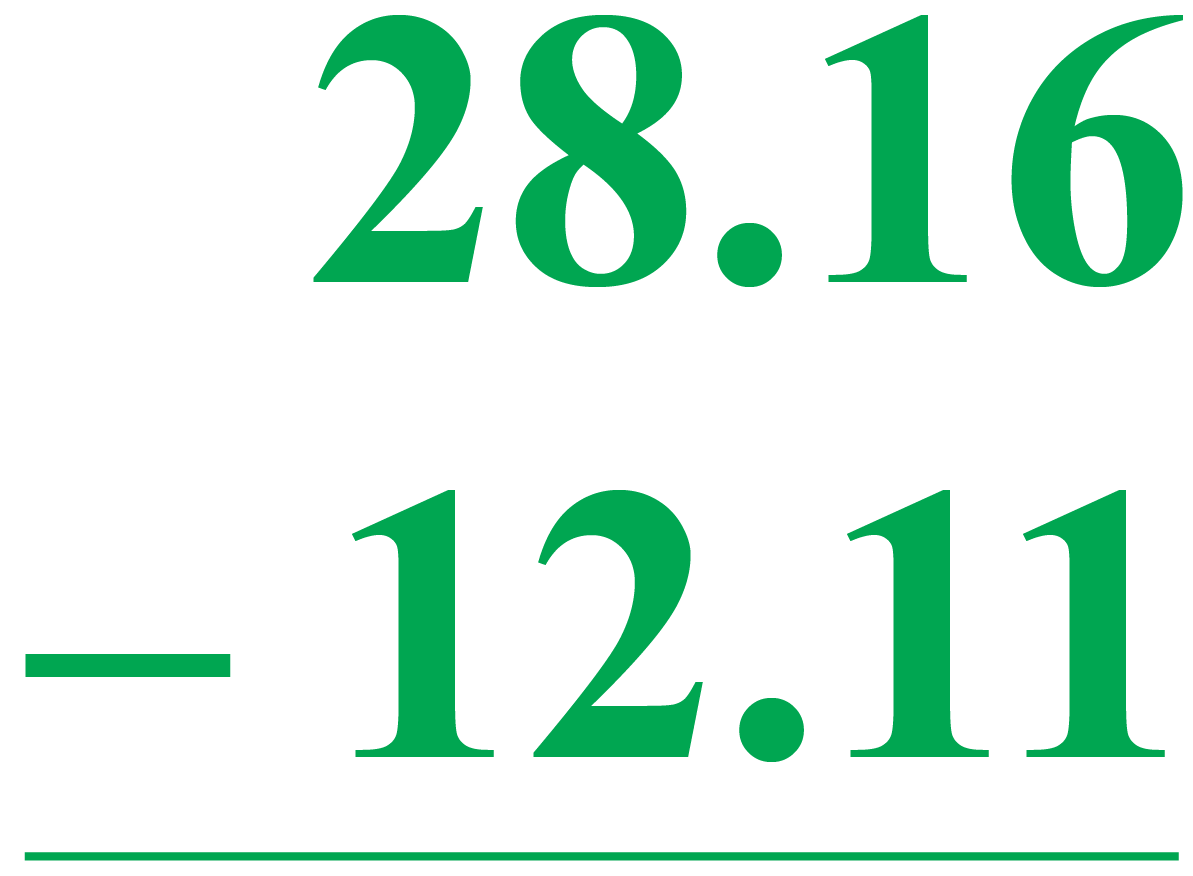• Step 2: Write zeroes in the places wherever the length of decimal numbers is not same.

This step does not apply in this case.

• Step 3: Now subtract the decimal numbers and find the output to subtraction.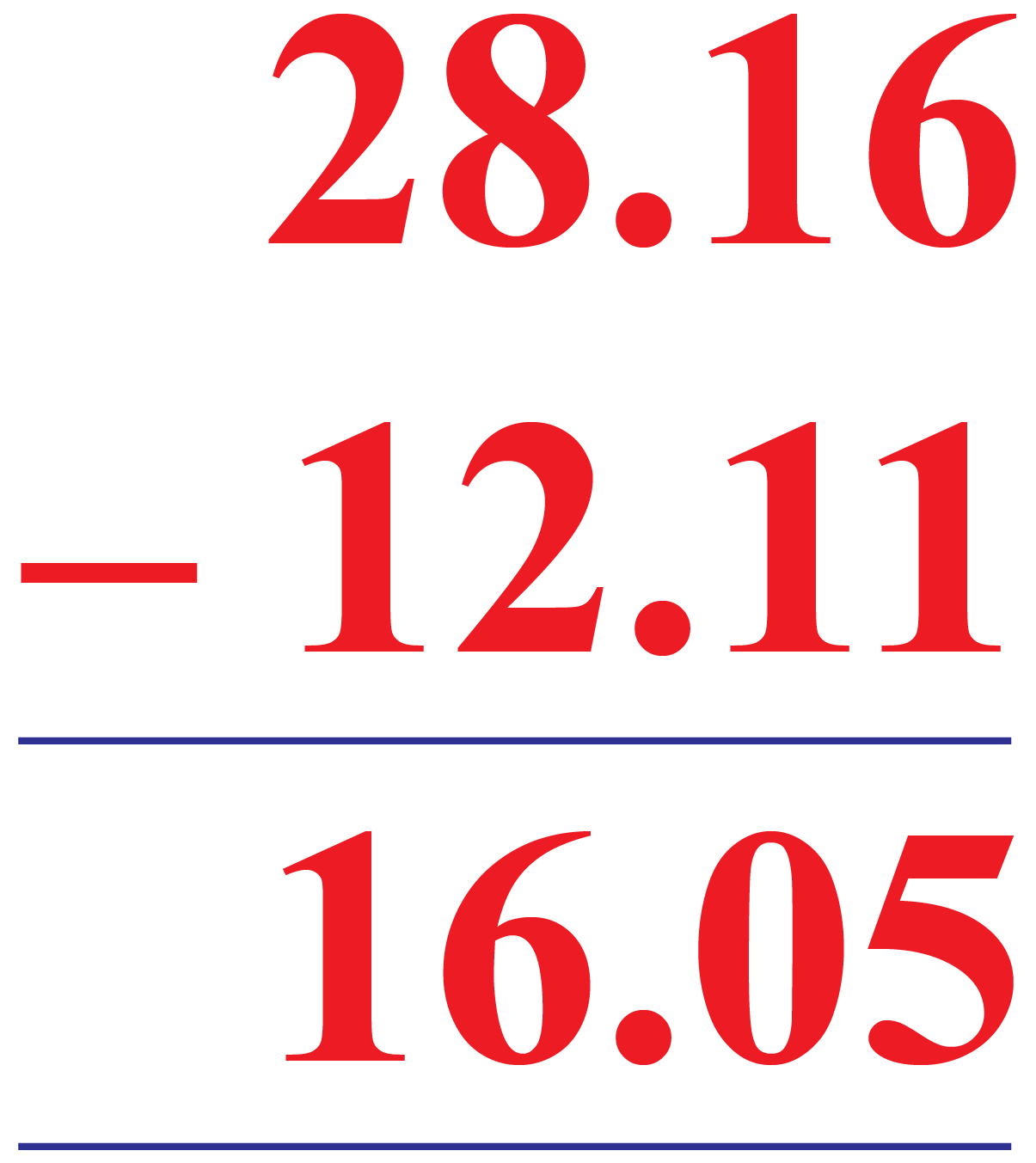The output obtained after subtraction of decimal numbers is, $$16.05$$.

### Subtract $$10.28$$ from $$21$$

• Step 1: Write the numbers, such that the decimals are lined perfectly.

The numbers will be written as follows: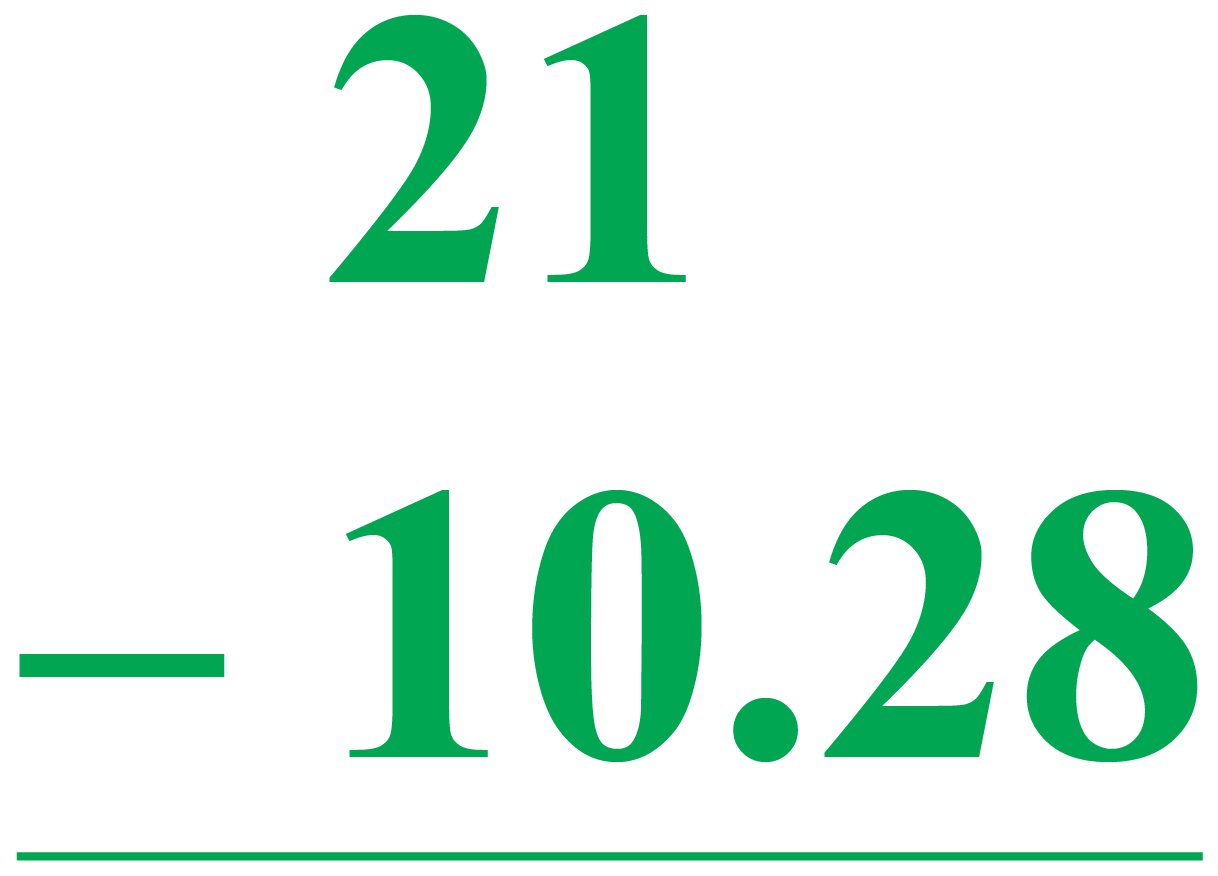• Step 2: Write zeroes in the places wherever the length of decimal numbers is not same.

This subtraction is written as: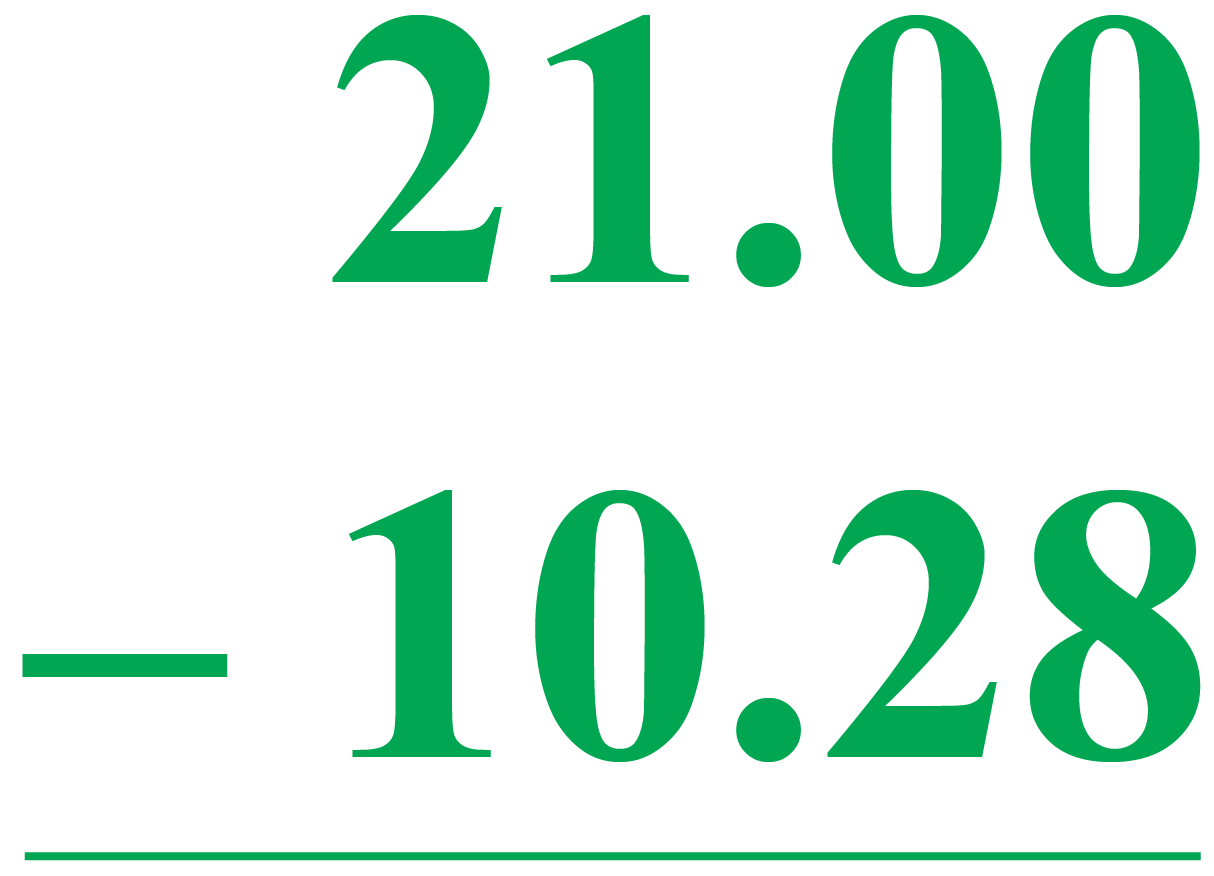• Step 3: Now subtract the decimal numbers and find the output to subtraction.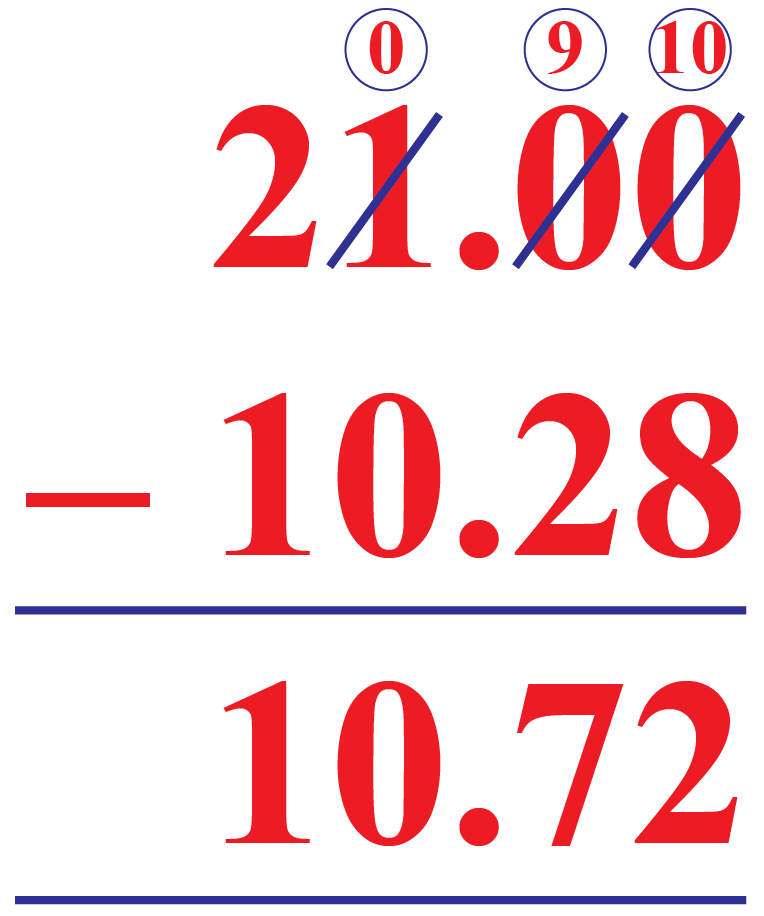The output obtained after subtraction of decimal numbers is, $$10.72$$

### Subtract $$10$$ from $$18.56$$

• Step 1: Write the numbers, such that the decimals are lined perfectly.

The numbers will be written as follows: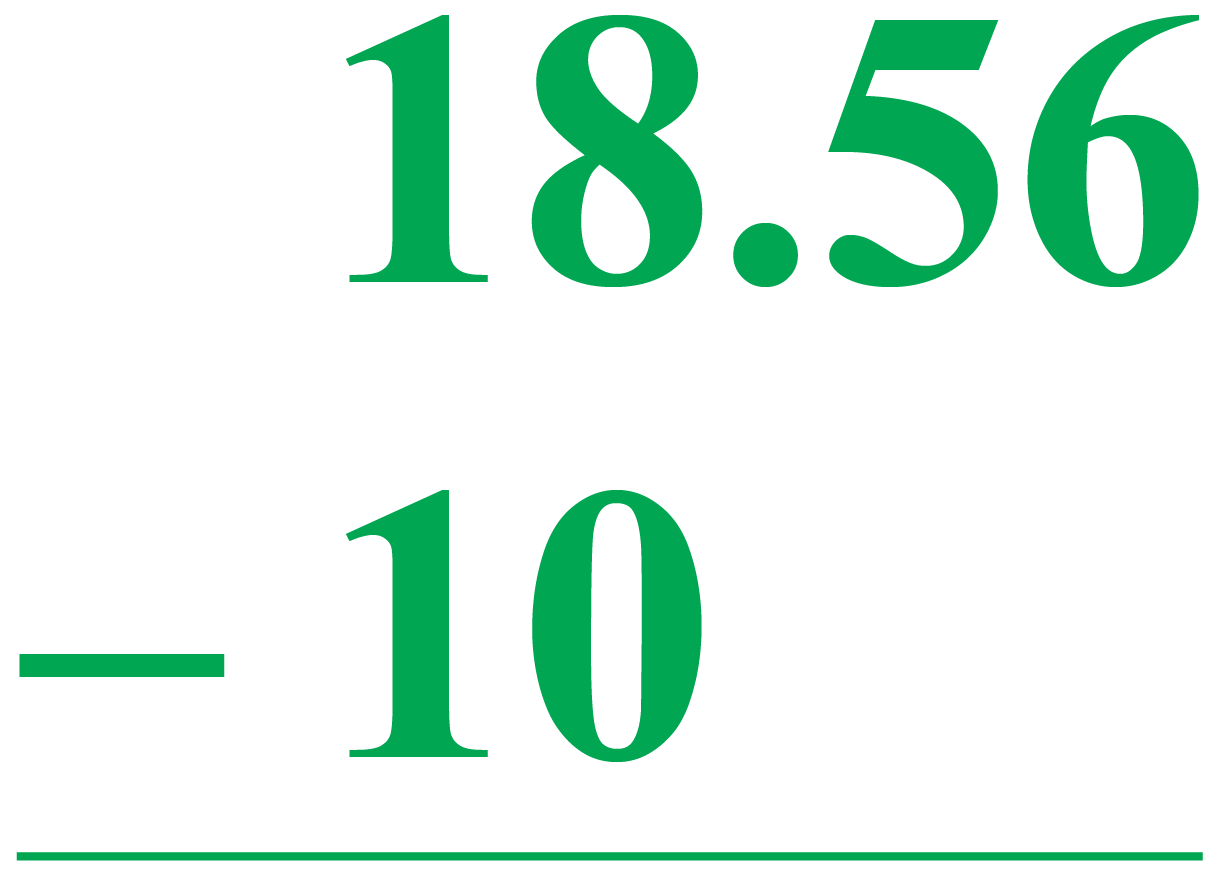• Step 2: Write zeroes in the places wherever the length of decimal numbers is not same.

This subtraction is written as: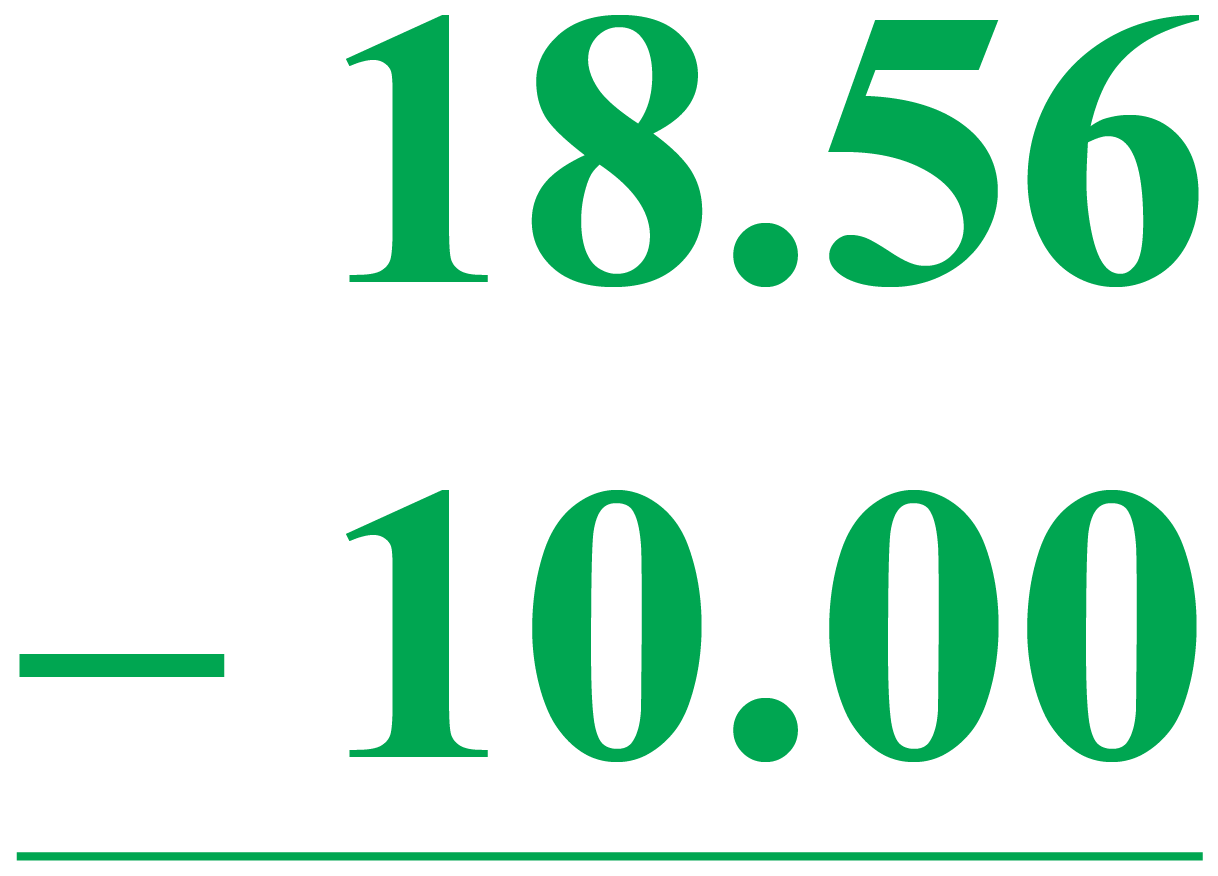• Step 3: Now subtract the decimal numbers and find the output to subtraction.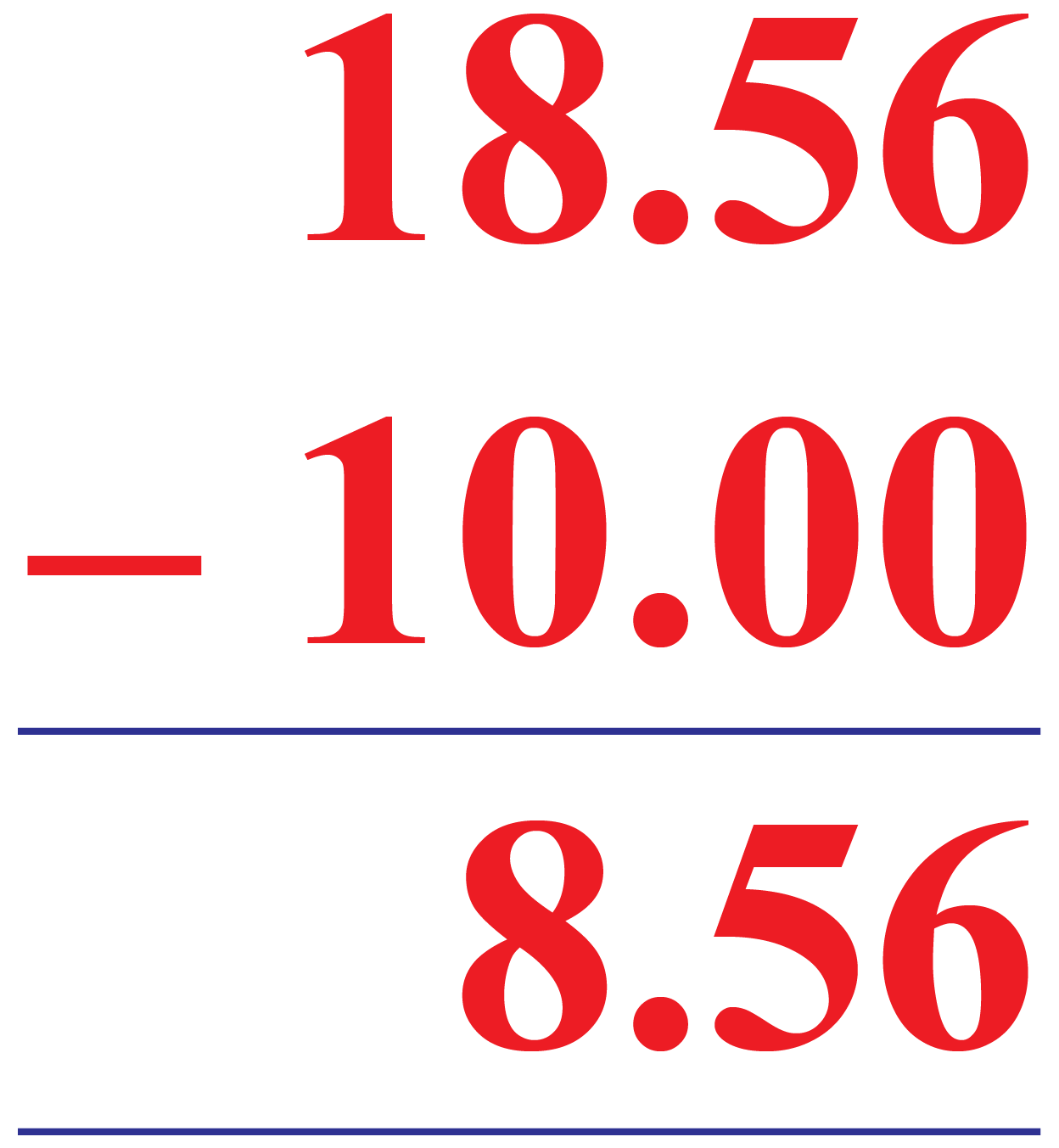The output obtained after subtraction of decimal numbers is, $$8.56$$

Let's have a look at the simulation below:

## How to Add Decimals with Fractions?

To add decimals with fractions following are the steps:

• Step 1: Convert the fraction into decimal.
• Step 2: Write the numbers, such that the decimals are lined perfectly.
• Step 3: Write zeroes in the places wherever the length of decimal numbers is not same.
• Step 4: Now add the decimal numbers and find the output to addition.

Let's have a look at few examples:

### Add $$7.28$$ to $$\dfrac{5}{8}$$

• Step 1: Convert the fraction into decimal.

The fraction $$\frac{5}{8}$$ as a decimal is written as $$0.625$$

• Step 2: Write the numbers, such that the decimals are lined perfectly.

The numbers will be written as follows: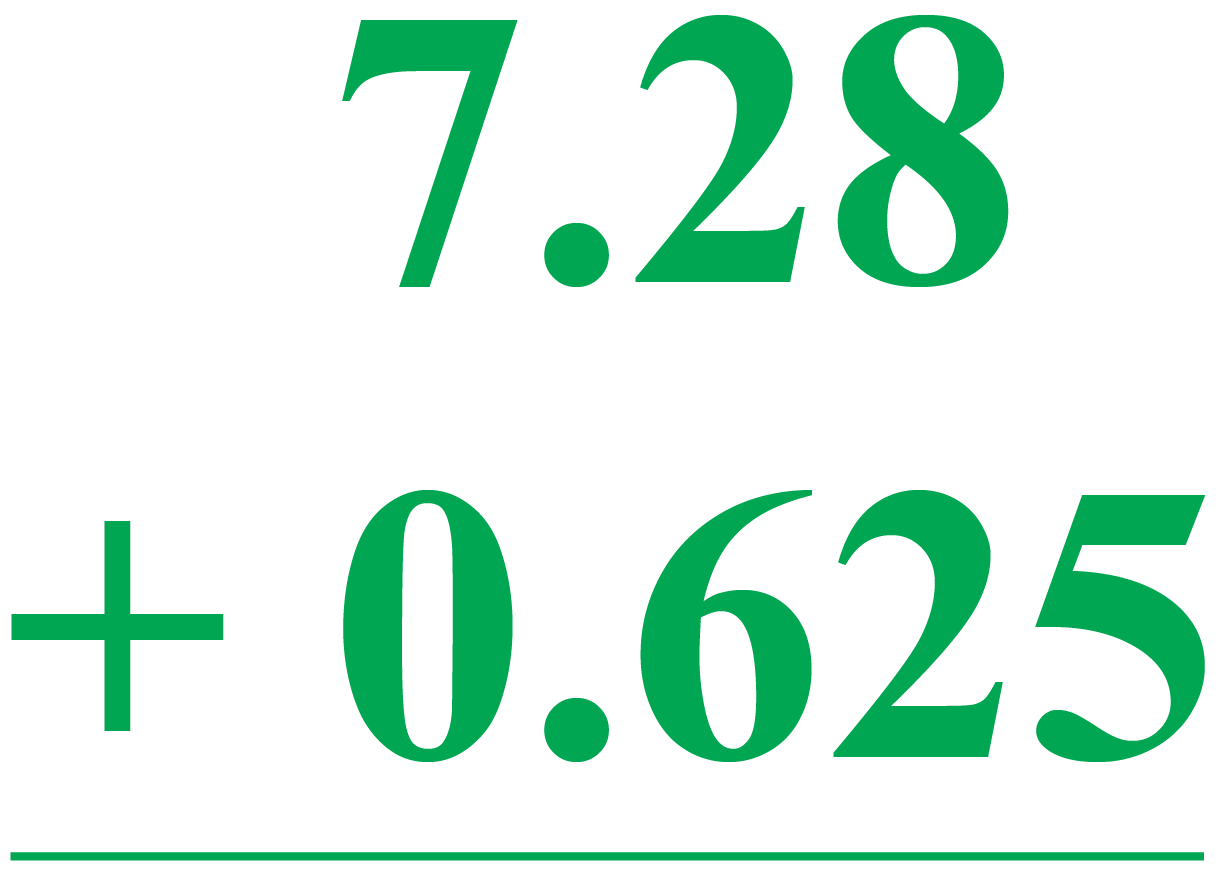• Step 3: Write zeroes in the places wherever the length of decimal numbers is not same.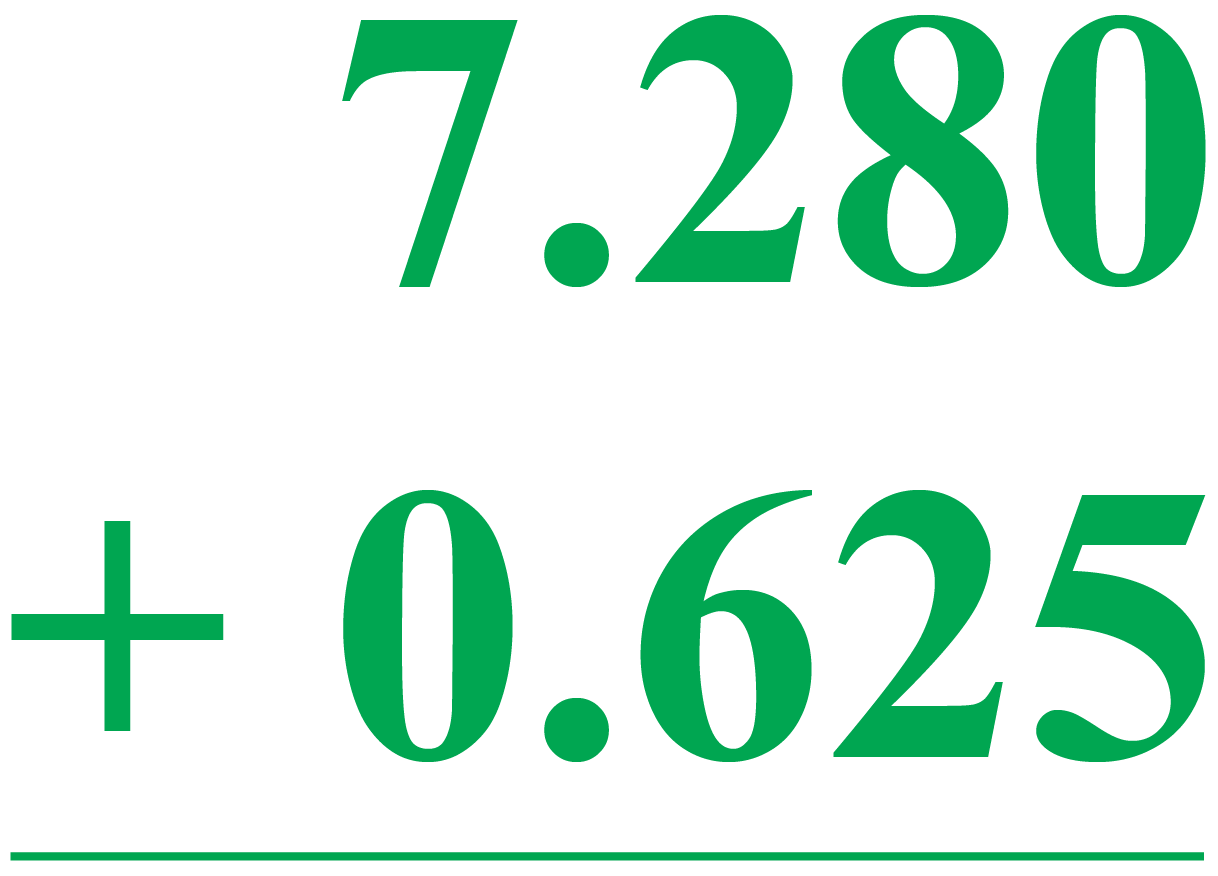• Step 4: Now add the decimal numbers and find the output to addition.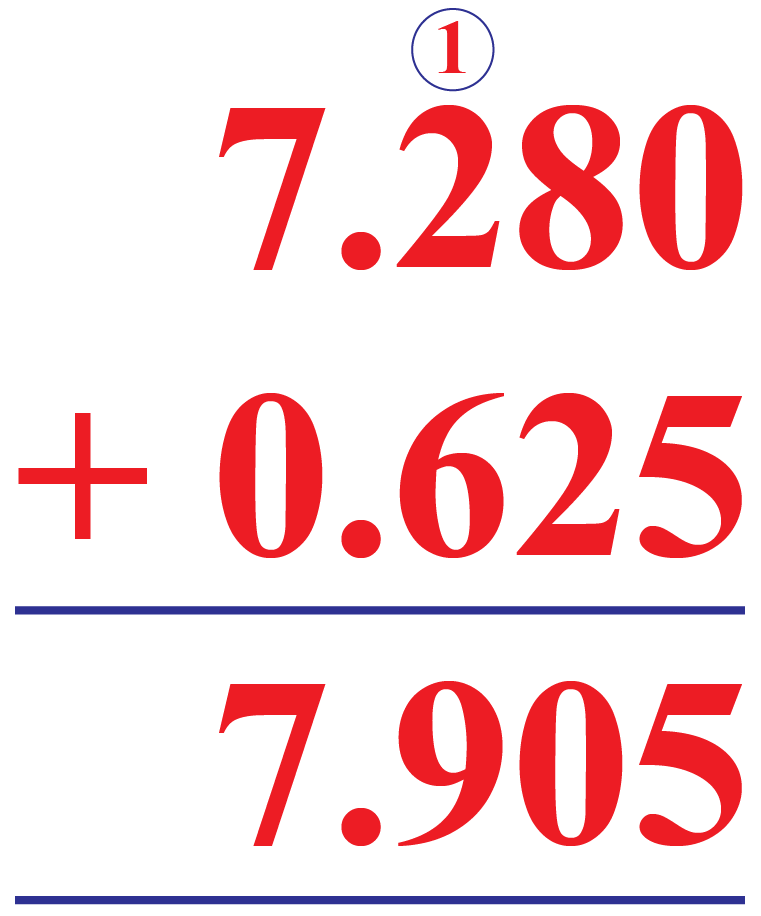The output obtained after addition of decimal number and fraction is, $$7.905$$.

### Add $$14.32$$ to $$\dfrac{1}{4}$$

• Step 1: Convert the fraction into decimal.

The fraction $$\frac{1}{4}$$ as a decimal is written as $$0.25$$

• Step 2: Write the numbers, such that the decimals are lined perfectly.

The numbers will be written as follows: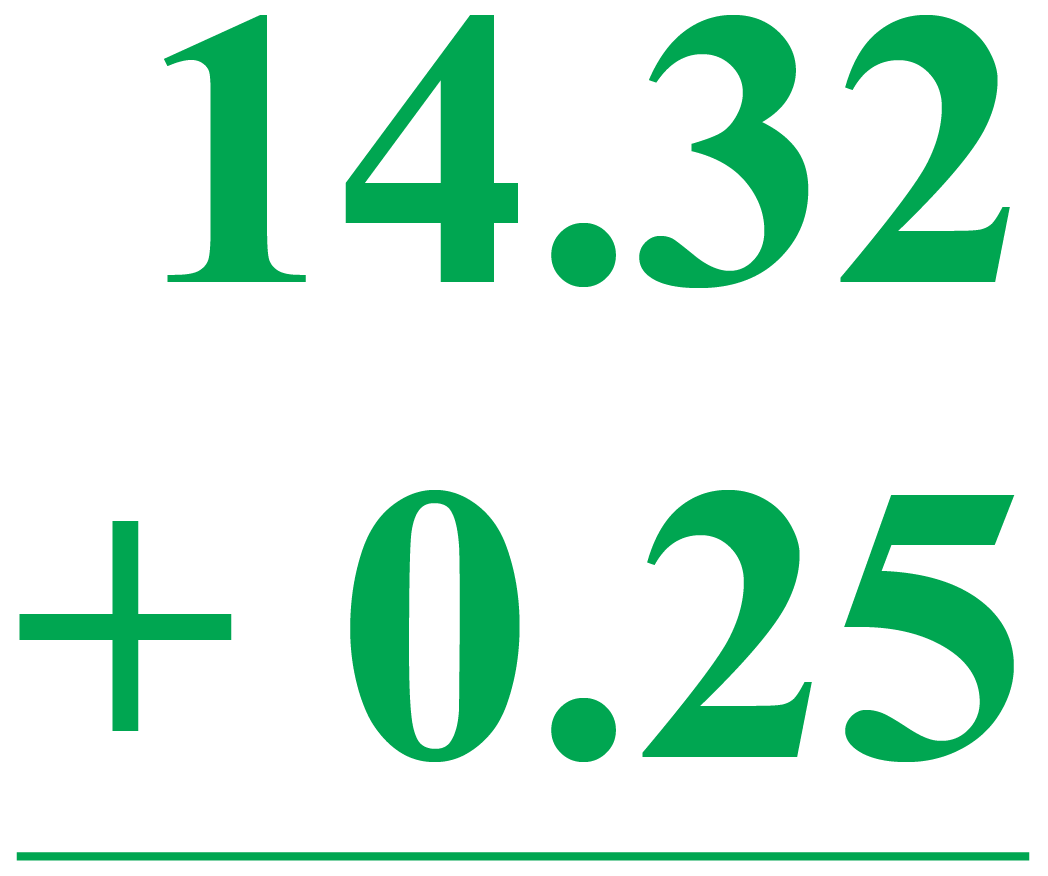• Step 3: Write zeroes in the places wherever the length of decimal numbers is not same.

This step does not apply here, as the length of number after the decimal is same.

• Step 4: Now add the decimal numbers and find the output to addition.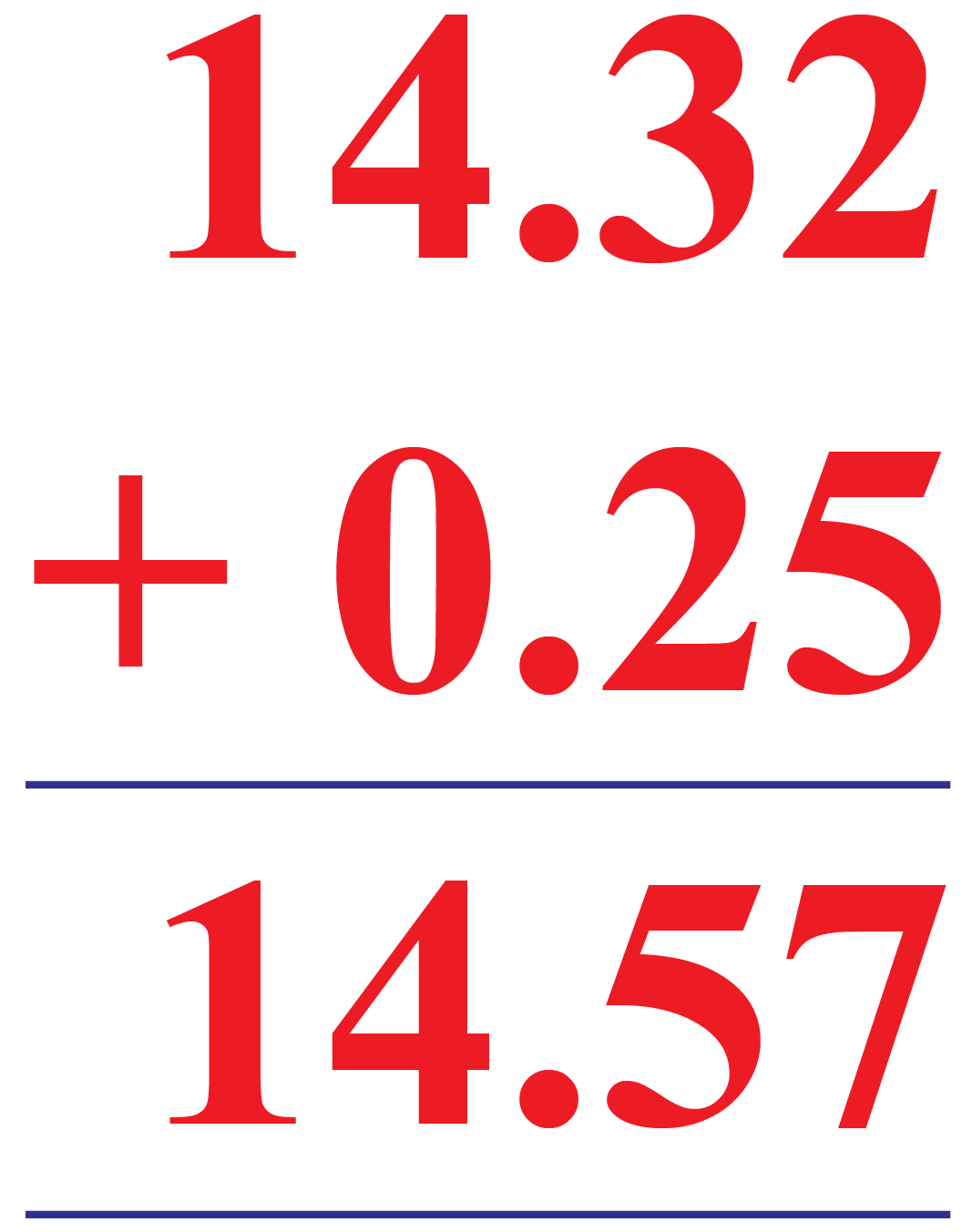The output obtained after addition of decimal number and fraction is, $$14.57$$

## Solved Examples

 Example 1

William and Wendy's teacher asked them to add 20.62 and 13.01 and find the answer . What is the answer they should get after addition of both the numbers?Solution

• Step 1: Write the numbers, such that the decimals are lined perfectly.

The numbers will be written as follows: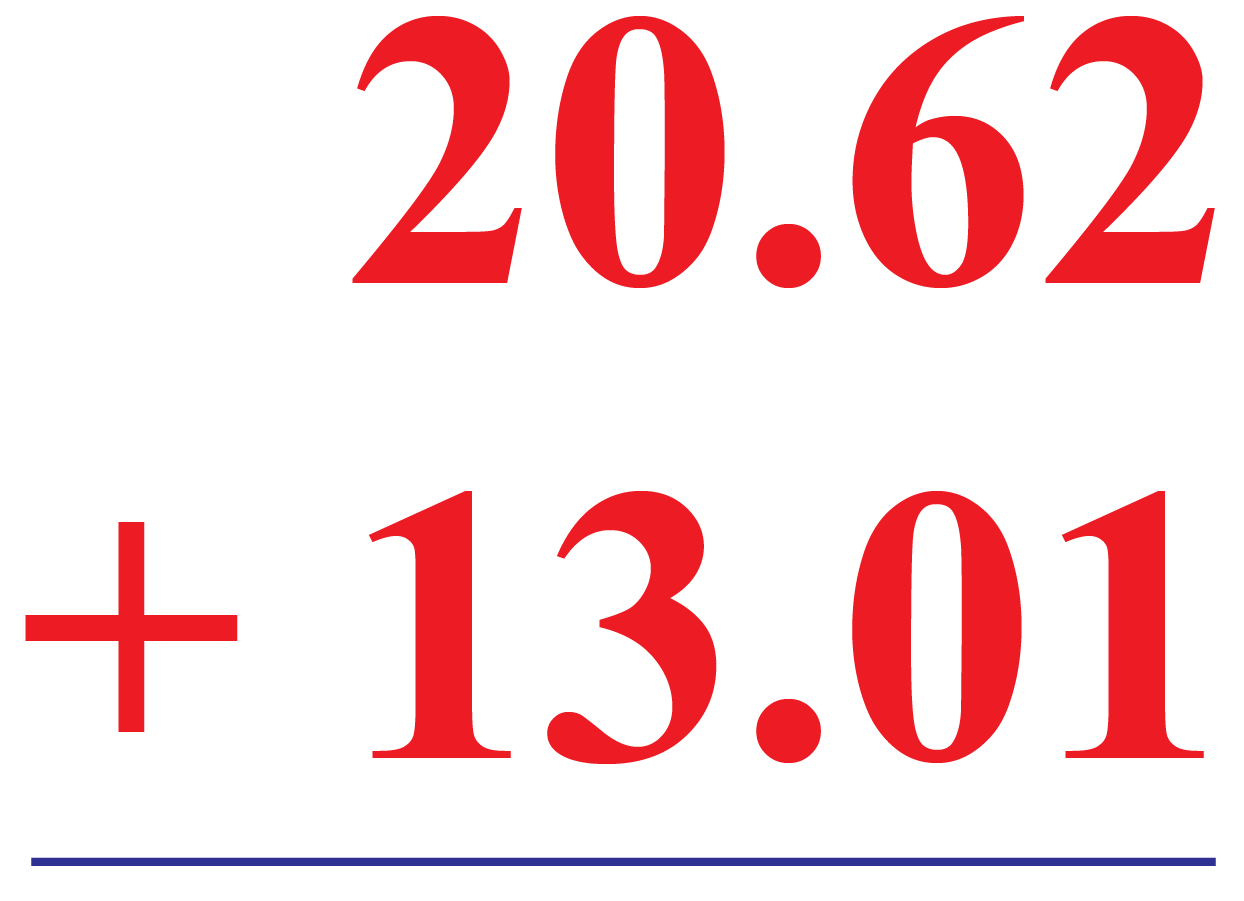• Step 2: Write zeroes in the places wherever the length of decimal numbers is not same.

This step does not apply in this case as length of the decimal numbers is same.

• Step 3: Now add the decimal numbers and find the output to addition.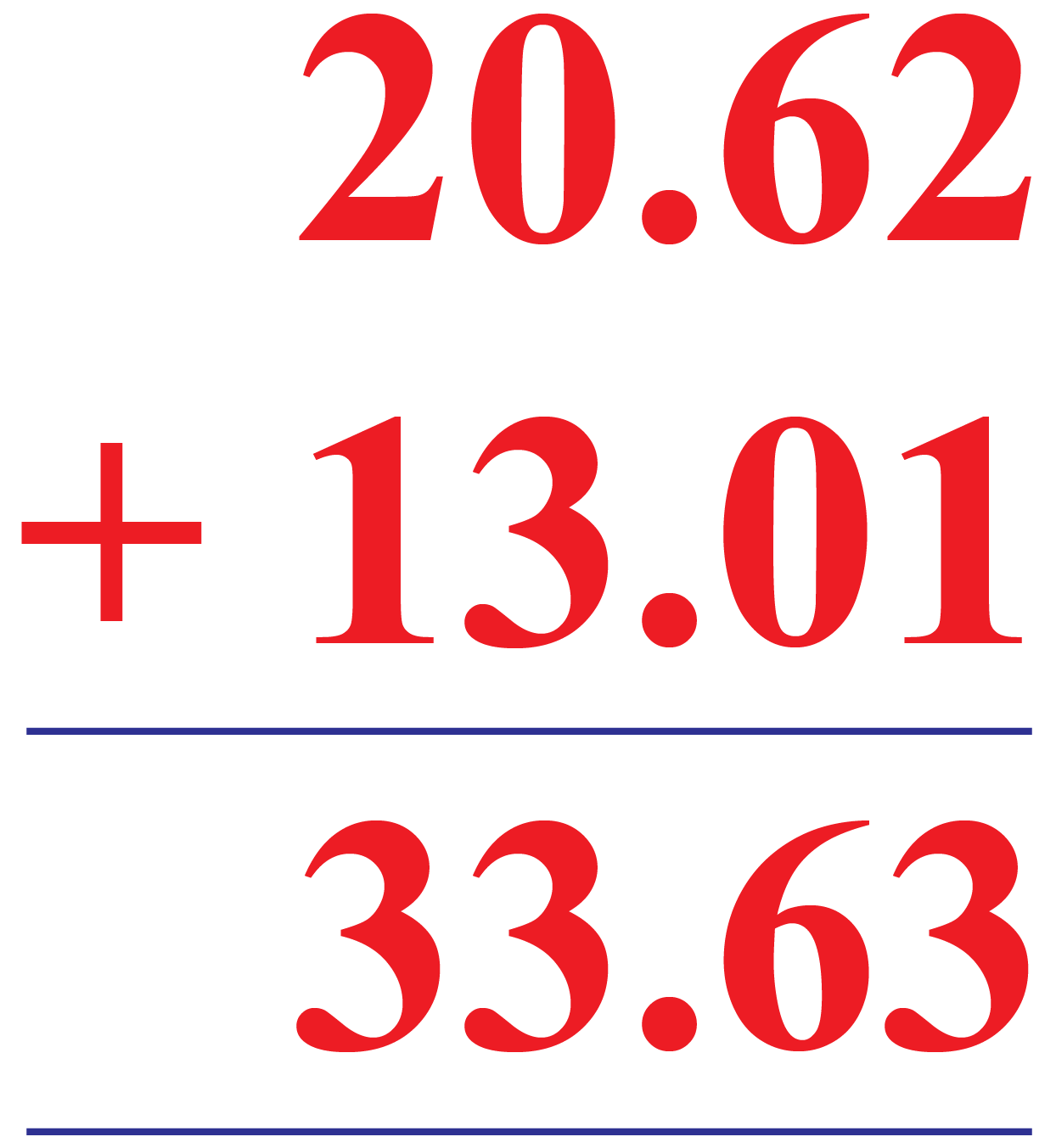The output obtained after addition of decimal numbers is, $$33.63$$

 $$\therefore$$ Answer is $$33.63$$
 Example 2

How will Shawn subtract 11.82 from 28.61? Help him find the answer.Solution

Shawn will use the following steps to obtain his answer:

• Step 1: Write the numbers, such that the decimals are lined perfectly.

The numbers will be written as follows: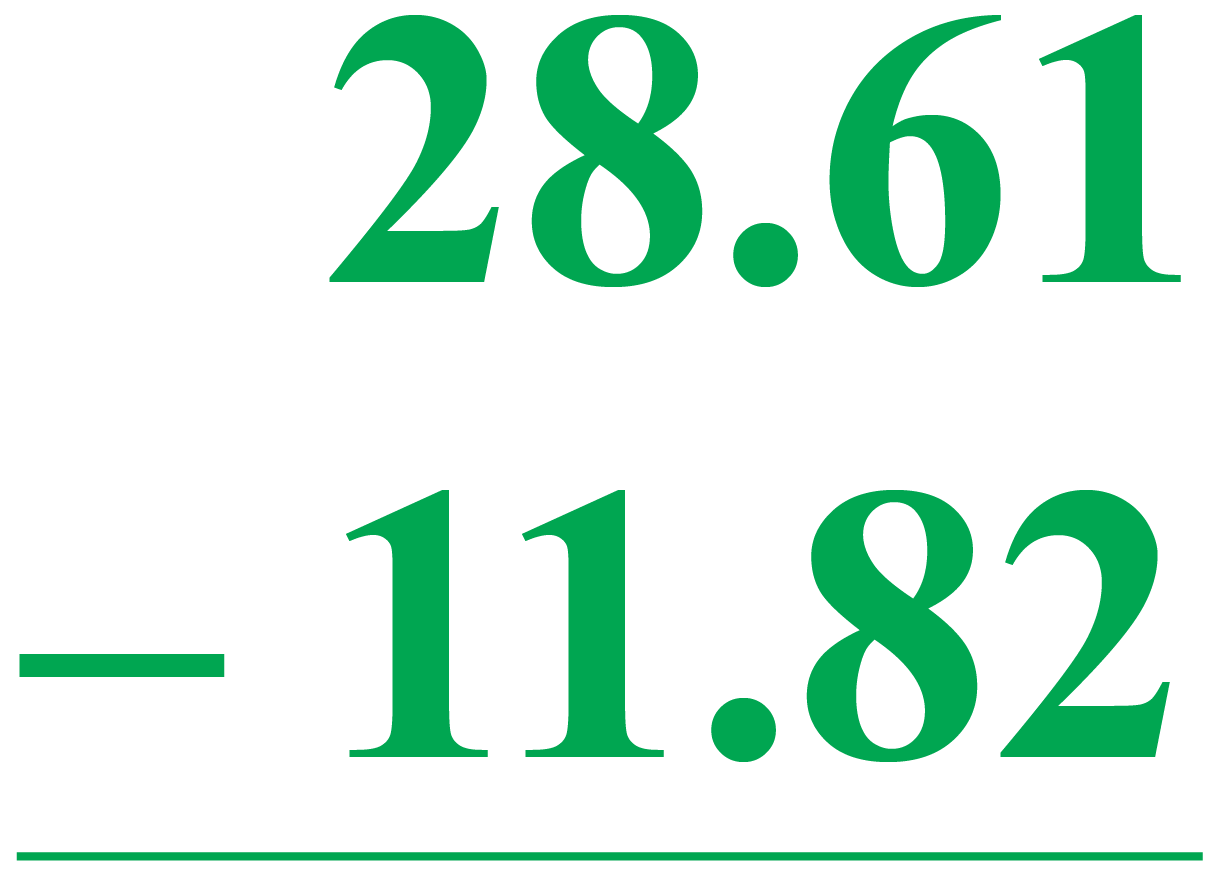• Step 2: Write zeroes in the places wherever the length of decimal numbers is not same.

This step does not apply in this case as length of the decimal numbers is same.

• Step 3: Now subtract the decimal numbers and find the output to subtraction.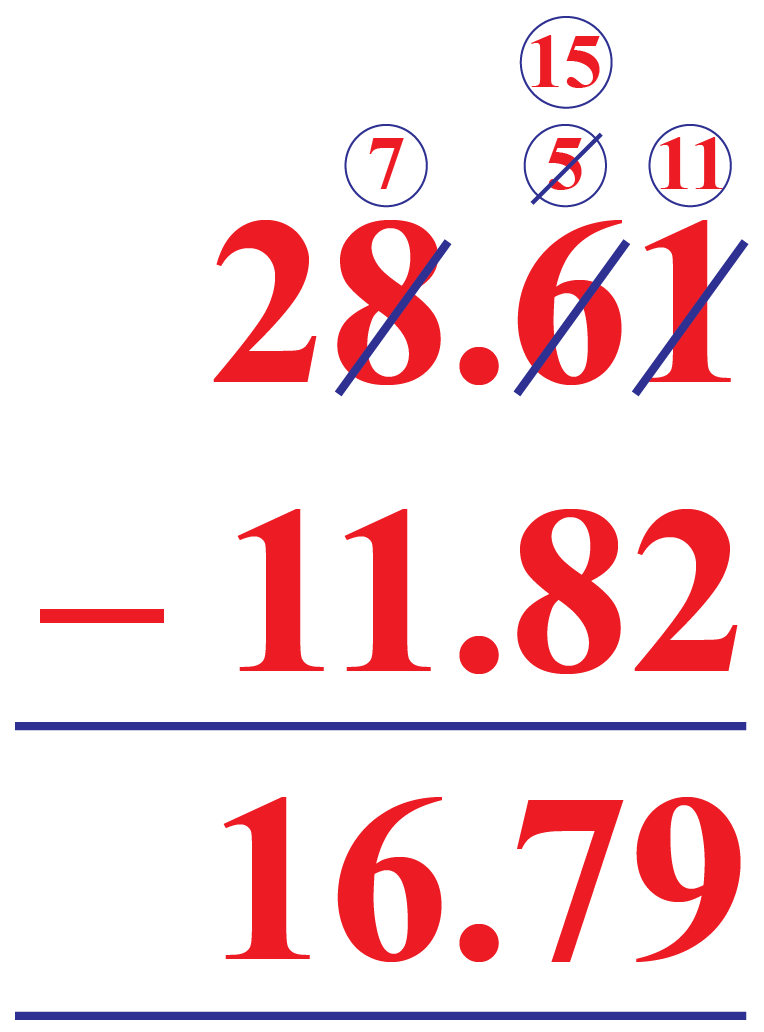The output obtained after addition of decimal numbers is, $$16.79$$

 $$\therefore$$ Answer is $$16.79$$

## Interactive Questions

Here are a few activities for you to practice.

## Let's Summarize

We hope you enjoyed learning about how to add decimals with the practice questions. Now, you will be able to easily find answers to questions like how to add and subtract decimals and how to add decimals with whole numbers.

At Cuemath, our team of math experts is dedicated to making learning fun for our favorite readers, the students!

Through an interactive and engaging learning-teaching-learning approach, the teachers explore all angles of a topic.

Be it worksheets, online classes, doubt sessions, or any other form of relation, it’s the logical thinking and smart learning approach that we at Cuemath believe in.

## 1. How to add and subtract decimals?

Step 1: Write the numbers, such that the decimals are lined perfectly.
Step 2: Write zeroes in the places wherever the length of decimal numbers is not same.
Step 3: Now add the decimal numbers and find the output to addition.

To subtract the decimals, we follow the below given steps:

Step 1: Write the numbers, such that the decimals are lined perfectly.
Step 2: Write zeroes in the places wherever the length of decimal numbers is not same.
Step 3: Now subtract the decimal numbers and find the output to subtraction.

## 2. How to add decimals with whole numbers?

To add decimals with whole numbers:

Step 1: Write the numbers, such that the decimals are lined perfectly.
Step 2: Put zeroes equal to the other decimal number length, which makes it a decimal number.
Step 3: Now add the decimal numbers and find the output to addition.

More Important Topics
Numbers
Algebra
Geometry
Measurement
Money
Data
Trigonometry
Calculus